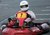## Dividing by 15 bug

A very helpful library, thank you for creating it.

One thing that confused me was the division by 15. It's not immediately obvious, but the data by default is in the highest 12 bits of a 16bit number spread across two registers. The sensitivity is expressed as X mg per digit, but this is per digit of the 12bit representation. Consequently, you need to right shift the 16bit representation by 4 (aka divide by 16, not 15).

I think this is a more obvious way of showing this in the code (from read_mg_data):

```    dt_usr = float(short((data << 8) | data) >> 4) * fs_factor;
dt_usr = float(short((data << 8) | data) >> 4) * fs_factor;
dt_usr = float(short((data << 8) | data) >> 4) * fs_factor;
```

.

#### Question relating to:LIS3DH / STMicroelectronics / MEMS motion sensor, 3-axis accelerometer library I2C, LIS3DH

posted by Allex Lmbed 16 Oct 2015

Hi Allex, there is actually a second bug here in that the sensitivity is expressed in g per digit, not mg per digit (e.g. #define LIS3DH_SENSITIVITY_2G (0.001F) where the sensitivity is 1mg per digit). So the function gives the results in g not mg. Example usage: float f = {0}; acc.read_mg_data(f); printf("acceleration in g is: a_x = %+.3f, a_y = %+.3f, a_z = %+.3f\r\n", f, f, f);

posted by Chris P 16 Oct 2015